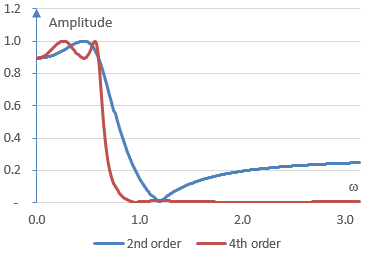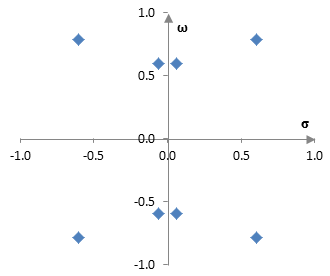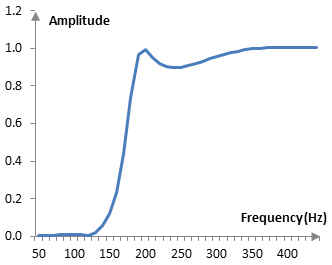# Elliptic filter

The low pass elliptic filter is defined by the gain function

$$G=\frac{1}{\sqrt{1+\epsilon^2\,R_n^2(\xi,\frac{\omega}{\omega_c})}}$$

where ω is the angular frequency, ωc is the cutoff frequency, ε and ξ are parameters that control the size of the ripples in the pass band and the stop band and Rn is the nth order elliptic rational function.

The elliptic filter is an equiripple filter, since the waves in its magnitude response are of equal height.

The elliptic rational function R does not have nice algebraic form, except in certain cases as described below. Hence, the elliptic filter is defined here with its gain function rather than with its transfer function. An example transfer function for one specific case is described below.

The function above is the gain function of the elliptic low pass filter. Changing s / ωc to ωc / s produces an elliptic high pass filter. Changing s / ωc to (s2 + ωc2) / (B s) produces the elliptic band pass filter, where ωc is the midpoint of the pass band and B is the width of the band. Changing s / ωc to B s / (s2 + ωc2) produces the elliptic band stop filter.

ε is called the ripple factor and controls the size of the ripples in the pass band. ξ is called the selectivity factor (ξ ≥ 1) and, together with the ripple factor, defines the size of the ripples in the stop band. As the ripple in the pass band approaches zero, the filter approaches the Chebychev filter of type I. As the ripple in the stop band approaches zero, the filter approaches the Chebychev filter of type II. As both ripples approach zero, the filter approaches the Butterworth filter.

The following is a plot of the gain function for orders 2 and 4 of the elliptic filter.In this particular example, ωc = 0.6, ε = 0.5, and ξ = 1.5. As ε approaches zero, the ripples in the pass band approaches zero. As ξ increases for a given ε, the ripple in the stop band approaches zero.

## An example second order elliptic filter

The second order elliptic rational function is

$$R_2(\xi,x)=\frac{(t+1)x^2-1}{(t-1)x^2+1}, \, t=\sqrt{1-\frac{1}{\xi^2}}$$

which means that the gain function of the second order low pass elliptic filter is

$$G=\frac{1}{\sqrt{1+\epsilon^2(\frac{(t+1)(\omega / \omega_x)^2-1}{(t-1)(\omega / \omega_c)^2+1})^2}}$$

and, for the Laplace transform transfer function H(s), with s = j ω, we have

$$H(js)H(-js)=\frac{1}{1+\epsilon^2(\frac{(t+1)(-s^2 / \omega_c^2)-1}{(t-1)(-s^2 / \omega_c^2)+1})^2}$$ $$=\frac{1}{1+\epsilon^2(\frac{(t+1)s^2+\omega_c^2}{(t-1)s^2-\omega_c^2})^2}$$

In the expression above, the four roots of the denominator are

$$s=\pm\omega_c(\frac{e^{\frac{j(2k-1)\pi}{2}}+\epsilon}{-\epsilon(t+1)+e^{\frac{j(2k-1)\pi}{2}}(t-1)})^{\frac{1}{2}},\,\,k=1,2$$

The transfer function can be written as

$$H(s)=\frac{1}{\sqrt{(t-1)^2+\epsilon^2(t+1)^2}} \frac{(t-1)s^2-\omega_c^2}{(s-s_1)(s-s_2)}$$

where s1 and s2 are the two roots, the real part of which is negative. (As with all infinite impulse response filters, we would like a filter that does not produce unbound output. Since we care only about positive amplitudes – there are no "negative amplitudes" in practice – there should be no roots in the denominator with positive real parts. Therefore, we only pick the roots with negative real parts.) After the bilinear transformation, s = 2 (z – 1) / (z + 1), the filter becomes

$$H(z)=\frac{1}{\sqrt{(t-1)^2+\epsilon^2(t+1)^2}}$$ $$\frac{(\omega_c^2-4(t-1))+(2\omega_c^2+8(t-1))z^{-1}+(\omega_c^2-4(t-1))z^{-2}}{(4-2(s_1+s_2)+s_1s_2)+(-8+2s_1s_2)z^{-1}+(4+2(s_1+s_2)+s_1s_2)z^{-2}}$$

s1 and s2 are complex conjugates, which means s1 s2 and s1 + s2 are real numbers and the Z transform transfer function above has only real coefficients.

With ωc = 0.6, ε = 0.5, and ξ = 1.5, and after scaling to obtain 1 in the beginning of the denominator, the transfer function is

$$H(z)=\frac{0.2466-0.2357z^{-1}+0.2466z^{-2}}{1-1.1572z^{-1}+0.4452z^{-2}}$$

(The cutoff frequency of the filter is technically ωc = 2 arctan(0.6/2) ≈ 0.583, due to the warping of the frequency domain by the bilinear transformation) The magnitude response of the filter is the same as the gain function for the second order filter in the graph above.

## The elliptic rational function

The elliptic rational function cannot always be written out in a nice algebraic way. Its general definition involves the Jacobi elliptic cosine function and the elliptic integral. In certain cases, specifically in the cases where the order n = 2i 3j for integer values of i and j, we have:

$$R_1(\xi,x)=x$$ $$R_2(\xi,x)=\frac{(t+1)x^2-1}{(t-1)x^2+1}$$ $$R_3(\xi,x)=x \frac{(1-x_1^2)(x^2-x_2^2)}{(1-x_2^2)(x^2-x_1^2)}$$ $$x_1^2=\frac{2\xi^2\sqrt{X}}{\sqrt{8\xi^2(\xi^2+1)+12X\xi^2-X^3-\sqrt{X^3}}}$$ $$x_2^2=\frac{\xi^2}{x_1^2}$$ $$X=\sqrt{4\xi^2+(4\xi^2(\xi^2-1))^{2/3}}$$

The nesting property of the elliptic rational function allows us to compute higher orders. The nesting property is

$$R_n(R_m(\xi,\xi),R_m(\xi,x))=R_{n,m}(\xi,x)$$

Thus,

$$R_4(\xi,x)=R_2(R_2(\xi,\xi),R_2(\xi,x))$$ $$R_6(\xi,x)=R_3(R_2(\xi,\xi),R_2(\xi,x))$$

and so on.

## Higher order elliptic filters

Higher order elliptic filters can be designed in a similar way. To the extent that the elliptic rational function can be written in a closed form, it may be possible to find the roots of the denominator of the elliptic filter transfer function deterministically. In all cases, one should be able to find the roots of the denominator numerically. The paragraphs below present an example of a fourth order high pass elliptic filter.

The fourth order elliptic rational function can be expressed as follows.

$$R_4(\xi,x)=\frac{(1+t)(1+\sqrt{t})^2 x^4-2(1+t)(1+\sqrt{t}) x^2 + 1}{(1+t)(1-\sqrt{t})^2 x^4-2(1+t)(1-\sqrt{t}) x^2 + 1}$$

The transfer function of the fourth order high pass elliptic filter then is

$$H^2(s)=\frac{1}{1+\epsilon^2\frac{(1+t)(1+\sqrt{t})^2 \frac{\omega_c^4}{s^4}-2(1+t)(1+\sqrt{t}) \frac{\omega_c^2}{-s^2} + 1}{(1+t)(1-\sqrt{t})^2 \frac{\omega_c^4}{s^4}-2(1+t)(1-\sqrt{t}) \frac{\omega_c^2}{-s^2} + 1}}$$

Set

$$a=2(1+t)(1+\sqrt{t})\omega_c^2$$ $$b=(1+t)(1+\sqrt{t})^2\omega_c^4$$ $$c=2(1+t)(1-\sqrt{t}) \omega_c^2$$ $$d=(1+t)(1-\sqrt{t})^2\omega_c^4$$

The eight roots of the denominator are as follows (two counts for all +- before each "j", which work together; two counts for the +- in front of the square root; and two counts the +- in the beginning).

$$s=\pm \frac{-(\epsilon a \pm j c) \pm \sqrt{(\epsilon a \pm j c)-4(\epsilon \pm j)(\epsilon b \pm jd)}}{2(\epsilon \pm j)}$$

Then the transfer function is

$$H(s)=\frac{1}{\sqrt{1+\epsilon^2}} \frac{s^4+c s^2+d}{(s-s_1)(s-s_2)(s-s_3)(s-s_4)}$$

where s1, s2, s3, and s4 are the four roots with negative real parts.

For example, with the same parameters as above (ωc = 0.6, ε = 0.5, and ξ = 1.5), the eight roots are shown below.The four roots with negative real parts are

$$s_1=-0.0632-0.5963j$$ $$s_2=-0.0632+0.5963j$$ $$s_3=-0.6012+0.7846j$$ $$s_4=-0.6012-0.7846j$$

The filter, after the bilinear transformation, is

$$H(z)=\frac{0.4835-1.6809z^{-1}+2.4866z^{-2}-1.6809z^{-3}+0.4835z^{-4}}{1-2.3976z^{-1}+2.5318z^{-2}-1.2794z^{-3}+0.3103z^{-4}}$$

The magnitude response of the filter is shown on the graph below.This graph was computed with the sampling frequency of 2000 Hz and, hence, the cutoff frequency of ωc = 0.6 translates to 191 Hz.

### Filtered HTML

• Freelinking helps you easily create HTML links. Links take the form of [[indicator:target|Title]]. By default (no indicator): Click to view a local node.